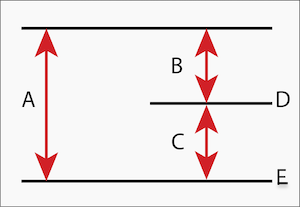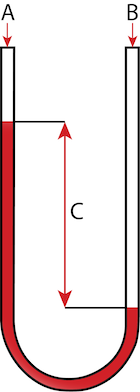# Differences between atmospheric, absolute, gauge, & differential pressureFigure 1: Visualization of the different pressure types: absolute pressure (A), gauge pressure (B), vacuum pressure (C), atmospheric pressure (D), and absolute zero pressure (E).

Pressure is a measure of force per unit area. There are different types of pressure measurements. There are two reference pressures: atmospheric and absolute zero pressure. Atmospheric pressure at sea level is 1.013 bar (14.7 psi). Absolute zero pressure is theoretically a perfect vacuum. A perfect vacuum’s conditions are impossible to create, even in interstellar space. This article discusses the differences between the types of pressure and pressure gauges used to measure them.

1. Absolute pressure (Pa) or (Pabs): Absolute pressure (Figure 1 labeled A) measures the pressure above absolute zero pressure (Figure 1 labeled E).
2. Differential (dp): Differential pressure is the difference between two different pressure measurements.
3. Gauge (Pg) or (Pgauge): Gauge pressure (Figure 1 labeled B) measures the pressure above atmospheric pressure (Figure 1 labeled D).
4. Vacuum (Pvac): Vacuum pressure (Figure 1 labeled C) measures the pressure below atmospheric pressure.
5. Atmospheric pressure (Patm): Pressure on the earth’s surface.

## 1) Absolute pressure

Because absolute pressure uses absolute zero pressure as a reference, it cannot have a negative value. Absolute pressure is the sum of gauge pressure and atmospheric pressure.

### Absolute pressure gauge

Absolute pressure gauges are applicable for industrial vacuum pumps. An absolute pressure gauge has a diaphragm, with one side contacting pressure media and the other connected to a vacuum. Therefore, atmospheric pressure does not influence the diaphragm’s deformation.

## 2) Differential pressureFigure 2: Differential pressure is a high-pressure reading (A) minus a low-pressure reading (B). The result on the pressure gauge is the difference between the two pressures (C).

Differential pressure is the difference between two applied pressures. Most pressure readings are technically differential as the pressure is measured relative to atmospheric pressure or absolute vacuum.

### Differential pressure gauge

A differential pressure gauge has two ports; one port connects to high pressure in the system, and one connects to low pressure. The differential pressure gauge simultaneously measures both pressures and displays the difference between them. A common use of a differential pressure gauge is to measure pressure drop across a system component, such as a valve.

## 3) Gauge pressure

Gauge pressure measures the difference between a system at the surrounding atmospheric pressure. Gauge pressure can be negative or positive, depending on whether the system is a vacuum.

Pressure gauges have a sensing element, such as a diaphragm, that deflects due to the pressure change. Pressure sensors require ventilation to use atmospheric pressure as their reference point. The gauge pressure is independent of the atmospheric pressure. It does not change with elevation, as the gauge pressure always takes local atmospheric pressure into account. Therefore, it is suitable for measuring pressure in pipes and tanks where pressure is higher than atmospheric pressure.

## 4) Vacuum pressure

Vacuum pressure is also known as negative gauge pressure. Pressure gauges read 0 at atmospheric pressure.

## 5) Atmospheric pressure

Atmospheric, or barometric, pressure is the force that air above a surface exerts on that surface. Atmospheric pressure lowers as altitude increases. At sea level, atmospheric pressure is 1.013 bar or 14.7 psi.

### Atmospheric pressure gauge

Today’s digital atmospheric and barometric pressure gauges provide highly precise data readouts. These gauges use micro-electromechanical sensors to measure pressure. There are two main types: capacitive and piezoresistive.

• Capacitive: Conductive sensors between a diaphragm create a capacitor. When the diaphragm deforms, the capacitance alters proportional to pressure.
• Piezoresistive: Similar to capacitive, except the conductive sensor is directly on the diaphragm.

## FAQs

### Can gauge pressure be negative?

Yes, gauge pressure can be negative. Gauge pressure is relative to atmospheric pressure. If measuring a vacuum, gauge pressure is negative.

### What is the difference between absolute pressure, atmospheric pressure, and gauge pressure?

Atmospheric pressure is the weight of the air on a surface and changes with altitude. Absolute pressure is pressure above absolute zero pressure, or a perfect vacuum. Gauge pressure is relative to atmospheric pressure.